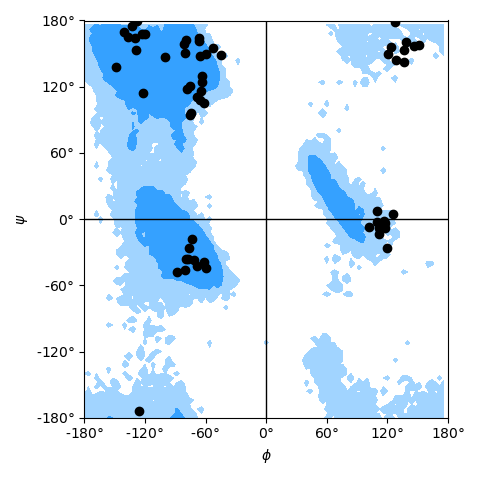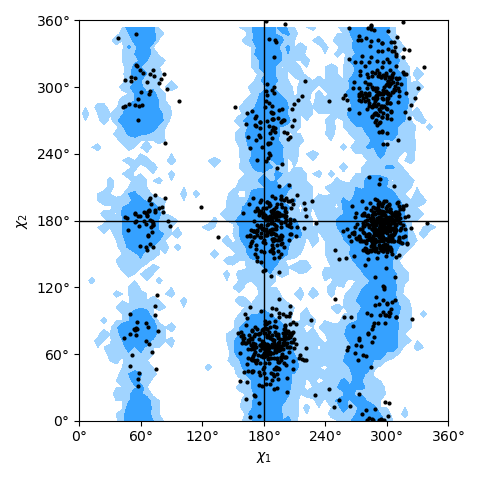# 4.7.1.3. Dihedral angles analysis — MDAnalysis.analysis.dihedrals¶

Author: Henry Mull 2018 GNU Public License v2

New in version 0.19.0.

This module contains classes for calculating dihedral angles for a given set of atoms or residues. This can be done for selected frames or whole trajectories.

A list of time steps that contain angles of interest is generated and can be easily plotted if desired. For the Ramachandran and Janin classes, basic plots can be generated using the method Ramachandran.plot() or Janin.plot(). These plots are best used as references, but they also allow for user customization.

MDAnalysis.lib.distances.calc_dihedrals()
function to calculate dihedral angles from atom positions

## 4.7.1.3.1. Example applications¶

### 4.7.1.3.1.1. General dihedral analysis¶

The Dihedral class is useful for calculating angles for many dihedrals of interest. For example, we can find the phi angles for residues 5-10 of adenylate kinase (AdK). The trajectory is included within the test data files:

import MDAnalysis as mda
from MDAnalysisTests.datafiles import GRO, XTC
u = mda.Universe(GRO, XTC)

# selection of atomgroups
ags = [res.phi_selection() for res in u.residues[4:9]]

from MDAnalysis.analysis.dihedrals import Dihedral
R = Dihedral(ags).run()


The angles can then be accessed with Dihedral.results.angles.

### 4.7.1.3.1.2. Ramachandran analysis¶

The Ramachandran class allows for the quick calculation of classical Ramachandran plots [Ramachandran1963] in the backbone $$phi$$ and $$psi$$ angles. Unlike the Dihedral class which takes a list of atomgroups, this class only needs a list of residues or atoms from those residues. The previous example can repeated with:

u = mda.Universe(GRO, XTC)
r = u.select_atoms("resid 5-10")

R = Ramachandran(r).run()


Then it can be plotted using the built-in plotting method Ramachandran.plot():

import matplotlib.pyplot as plt
fig, ax = plt.subplots(figsize=plt.figaspect(1))
R.plot(ax=ax, color='k', marker='o', ref=True)
fig.tight_layout()


as shown in the example Ramachandran plot figure.Ramachandran plot for residues 5 to 10 of AdK, sampled from the AdK test trajectory (XTC). The contours in the background are the “allowed region” and the “marginally allowed” regions.

To plot the data yourself, the angles can be accessed using Ramachandran.results.angles.

Note

The Ramachandran analysis is prone to errors if the topology contains duplicate or missing atoms (e.g. atoms with altloc or incomplete residues). If the topology has as an altloc attribute, you must specify only one altloc for the atoms with more than one ("protein and not altloc B").

### 4.7.1.3.1.3. Janin analysis¶

Janin plots [Janin1978] for side chain conformations ($$\chi_1$$ and $$chi_2$$ angles) can be created with the Janin class. It works in the same way, only needing a list of residues; see the Janin plot figure as an example.

The data for the angles can be accessed in the attribute Janin.results.angles.Janin plot for all residues of AdK, sampled from the AdK test trajectory (XTC). The contours in the background are the “allowed region” and the “marginally allowed” regions for all possible residues.

Note

The Janin analysis is prone to errors if the topology contains duplicate or missing atoms (e.g. atoms with altloc or incomplete residues). If the topology has as an altloc attribute, you must specify only one altloc for the atoms with more than one ("protein and not altloc B").

Furthermore, many residues do not have a $$\chi_2$$ dihedral and if the selections of residues is not carefully filtered to only include those residues with both sidechain dihedrals then a ValueError with the message Too many or too few atoms selected is raised.

### 4.7.1.3.1.4. Reference plots¶

Reference plots can be added to the axes for both the Ramachandran and Janin classes using the kwarg ref=True for the Ramachandran.plot() and Janin.plot() methods. The Ramachandran reference data (Rama_ref) and Janin reference data (Janin_ref) were made using data obtained from a large selection of 500 PDB files, and were analyzed using these classes [Mull2018]. The allowed and marginally allowed regions of the Ramachandran reference plot have cutoffs set to include 90% and 99% of the data points, and the Janin reference plot has cutoffs for 90% and 98% of the data points. The list of PDB files used for the reference plots was taken from [Lovell2003] and information about general Janin regions was taken from [Janin1978].

## 4.7.1.3.2. Analysis Classes¶

class MDAnalysis.analysis.dihedrals.Dihedral(atomgroups, **kwargs)[source]

Calculate dihedral angles for specified atomgroups.

Dihedral angles will be calculated for each atomgroup that is given for each step in the trajectory. Each AtomGroup must contain 4 atoms.

Note

This class takes a list as an input and is most useful for a large selection of atomgroups. If there is only one atomgroup of interest, then it must be given as a list of one atomgroup.

Changed in version 2.0.0: angles results are now stored in a MDAnalysis.analysis.base.Results instance.

Parameters: atomgroups (list) – a list of atomgroups for which the dihedral angles are calculated ValueError – If any atomgroups do not contain 4 atoms
results.angles

Contains the time steps of the angles for each atomgroup in the list as an n_frames×len(atomgroups) numpy.ndarray with content [[angle 1, angle 2, ...], [time step 2], ...].

New in version 2.0.0.

angles

Alias to the results.angles attribute.

Deprecated since version 2.0.0: Will be removed in MDAnalysis 3.0.0. Please use results.angles instead.

run(start=None, stop=None, step=None, verbose=None)

Perform the calculation

Parameters: start (int, optional) – start frame of analysis stop (int, optional) – stop frame of analysis step (int, optional) – number of frames to skip between each analysed frame verbose (bool, optional) – Turn on verbosity
class MDAnalysis.analysis.dihedrals.Ramachandran(atomgroup, c_name='C', n_name='N', ca_name='CA', check_protein=True, **kwargs)[source]

Calculate $$\phi$$ and $$\psi$$ dihedral angles of selected residues.

$$\phi$$ and $$\psi$$ angles will be calculated for each residue corresponding to atomgroup for each time step in the trajectory. A ResidueGroup is generated from atomgroup which is compared to the protein to determine if it is a legitimate selection.

Parameters: atomgroup (AtomGroup or ResidueGroup) – atoms for residues for which $$\phi$$ and $$\psi$$ are calculated c_name (str (optional)) – name for the backbone C atom n_name (str (optional)) – name for the backbone N atom ca_name (str (optional)) – name for the alpha-carbon atom check_protein (bool (optional)) – whether to raise an error if the provided atomgroup is not a subset of protein atoms

Example

For standard proteins, the default arguments will suffice to run a Ramachandran analysis:

r = Ramachandran(u.select_atoms('protein')).run()


For proteins with non-standard residues, or for calculating dihedral angles for other linear polymers, you can switch off the protein checking and provide your own atom names in place of the typical peptide backbone atoms:

r = Ramachandran(u.atoms, c_name='CX', n_name='NT', ca_name='S',
check_protein=False).run()


The above analysis will calculate angles from a “phi” selection of CX’-NT-S-CX and “psi” selections of NT-S-CX-NT’.

Raises: ValueError – If the selection of residues is not contained within the protein and check_protein is True

Note

If check_protein is True and the residue selection is beyond the scope of the protein and, then an error will be raised. If the residue selection includes the first or last residue, then a warning will be raised and they will be removed from the list of residues, but the analysis will still run. If a $$\phi$$ or $$\psi$$ selection cannot be made, that residue will be removed from the analysis.

Changed in version 1.0.0: added c_name, n_name, ca_name, and check_protein keyword arguments

Changed in version 2.0.0: angles results are now stored in a MDAnalysis.analysis.base.Results instance.

results.angles

Contains the time steps of the $$\phi$$ and $$\psi$$ angles for each residue as an n_frames×n_residues×2 numpy.ndarray with content [[[phi, psi], [residue 2], ...], [time step 2], ...].

New in version 2.0.0.

angles

Alias to the results.angles attribute.

Deprecated since version 2.0.0: Will be removed in MDAnalysis 3.0.0. Please use results.angles instead.

plot(ax=None, ref=False, **kwargs)[source]

Plots data into standard Ramachandran plot.

Each time step in Ramachandran.results.angles is plotted onto the same graph.

Parameters: ax (matplotlib.axes.Axes) – If no ax is supplied or set to None then the plot will be added to the current active axes. ref (bool, optional) – Adds a general Ramachandran plot which shows allowed and marginally allowed regions kwargs (optional) – All other kwargs are passed to matplotlib.pyplot.scatter(). ax – Axes with the plot, either ax or the current axes. matplotlib.axes.Axes
run(start=None, stop=None, step=None, verbose=None)

Perform the calculation

Parameters: start (int, optional) – start frame of analysis stop (int, optional) – stop frame of analysis step (int, optional) – number of frames to skip between each analysed frame verbose (bool, optional) – Turn on verbosity
class MDAnalysis.analysis.dihedrals.Janin(atomgroup, select_remove='resname ALA CYS* GLY PRO SER THR VAL', select_protein='protein', **kwargs)[source]

Calculate $$\chi_1$$ and $$\chi_2$$ dihedral angles of selected residues.

$$\chi_1$$ and $$\chi_2$$ angles will be calculated for each residue corresponding to atomgroup for each time step in the trajectory. A ResidueGroup is generated from atomgroup which is compared to the protein to determine if it is a legitimate selection.

Note

If the residue selection is beyond the scope of the protein, then an error will be raised. If the residue selection includes the residues ALA, CYS*, GLY, PRO, SER, THR, or VAL (the default of the select_remove keyword argument) then a warning will be raised and they will be removed from the list of residues, but the analysis will still run. Some topologies have altloc attribues which can add duplicate atoms to the selection and must be removed.

Parameters: atomgroup (AtomGroup or ResidueGroup) – atoms for residues for which $$\chi_1$$ and $$\chi_2$$ are calculated select_remove (str) – selection string to remove residues that do not have $$chi_2$$ angles select_protein (str) – selection string to subselect protein-only residues from atomgroup to check that only amino acids are selected; if you have non-standard amino acids then adjust this selection to include them ValueError – if the final selection of residues is not contained within the protein (as determined by atomgroup.select_atoms(select_protein)) ValueError – if not enough or too many atoms are found for a residue in the selection, usually due to missing atoms or alternative locations, or due to non-standard residues

Changed in version 2.0.0: select_remove and select_protein keywords were added. angles results are now stored in a MDAnalysis.analysis.base.Results instance.

results.angles

Contains the time steps of the $$\chi_1$$ and $$\chi_2$$ angles for each residue as an n_frames×n_residues×2 numpy.ndarray with content [[[chi1, chi2], [residue 2], ...], [time step 2], ...].

New in version 2.0.0.

angles

Alias to the results.angles attribute.

Deprecated since version 2.0.0: Will be removed in MDAnalysis 3.0.0. Please use results.angles instead.

plot(ax=None, ref=False, **kwargs)[source]

Plots data into standard Janin plot.

Each time step in Janin.results.angles is plotted onto the same graph.

Parameters: ax (matplotlib.axes.Axes) – If no ax is supplied or set to None then the plot will be added to the current active axes. ref (bool, optional) – Adds a general Janin plot which shows allowed and marginally allowed regions kwargs (optional) – All other kwargs are passed to matplotlib.pyplot.scatter(). ax – Axes with the plot, either ax or the current axes. matplotlib.axes.Axes
run(start=None, stop=None, step=None, verbose=None)

Perform the calculation

Parameters: start (int, optional) – start frame of analysis stop (int, optional) – stop frame of analysis step (int, optional) – number of frames to skip between each analysed frame verbose (bool, optional) – Turn on verbosity

References

 [Ramachandran1963] G. Ramachandran, C. Ramakrishnan, and V. Sasisekharan. (1963) Stereochemistry of polypeptide chain configurations. Journal of Molecular Biology, 7(1):95 – 99. doi: 10.1016/S0022-2836(63)80023-6
 [Janin1978] (1, 2) Joël Janin, Shoshanna Wodak, Michael Levitt, and Bernard Maigret. (1978). “Conformation of amino acid side-chains in proteins”. Journal of Molecular Biology 125(3): 357-386. doi: 10.1016/0022-2836(78)90408-4
 [Lovell2003] Simon C. Lovell, Ian W. Davis, W. Bryan Arendall III, Paul I. W. de Bakker, J. Michael Word, Michael G. Prisant, Jane S. Richardson, and David C. Richardson (2003). “Structure validation by $$C_{\alpha}$$ geometry: $$\phi$$, $$\psi$$, and $$C_{\beta}$$ deviation”. Proteins 50(3): 437-450. doi: 10.1002/prot.10286
 [Mull2018] H. Mull and O. Beckstein. SPIDAL Summer REU 2018 Dihedral Analysis in MDAnalysis. Technical report, Arizona State University, 8 2018. doi: 10.6084/m9.figshare.6957296